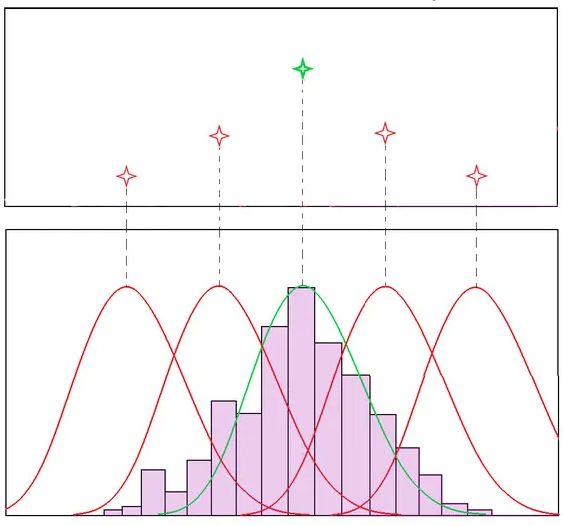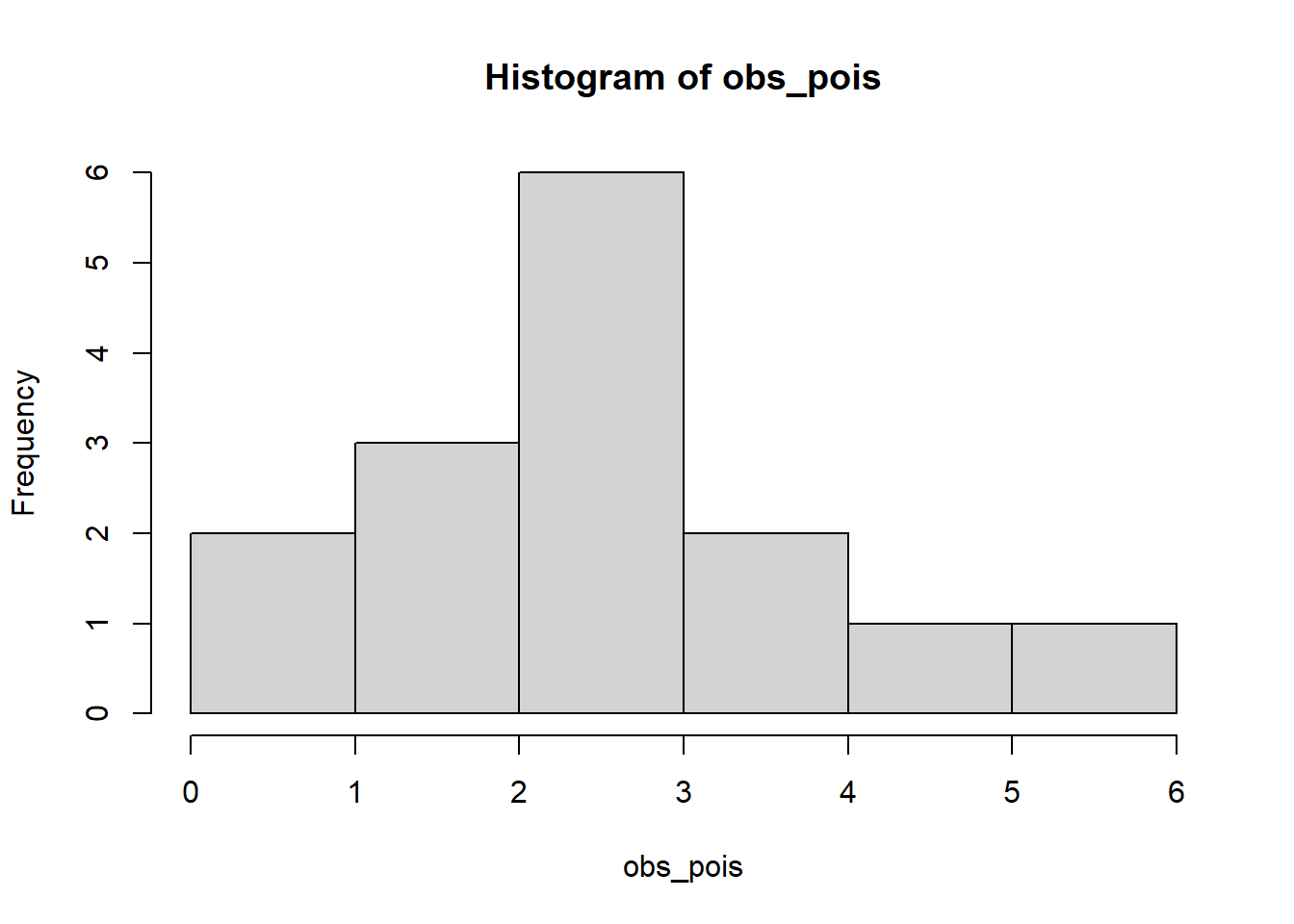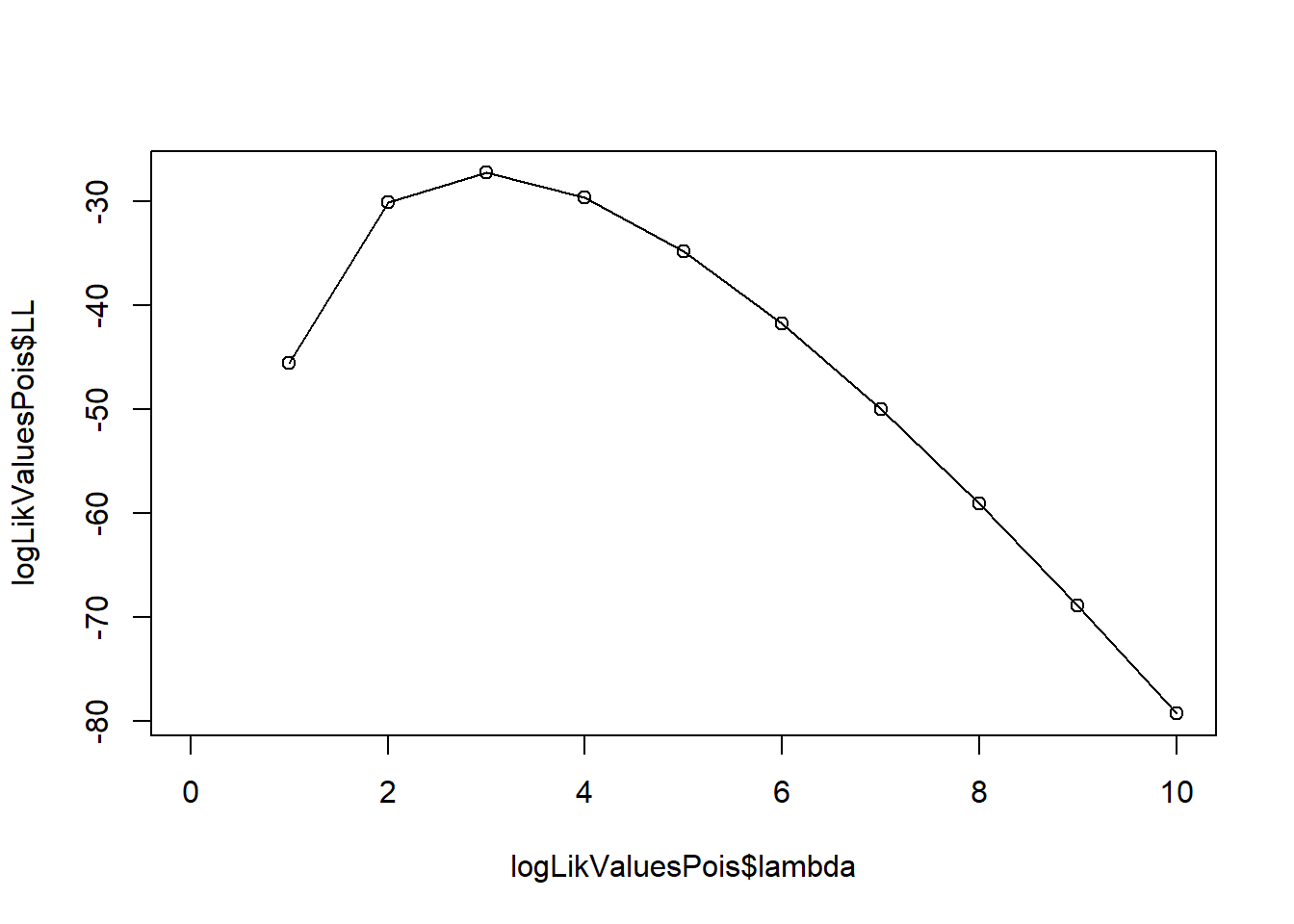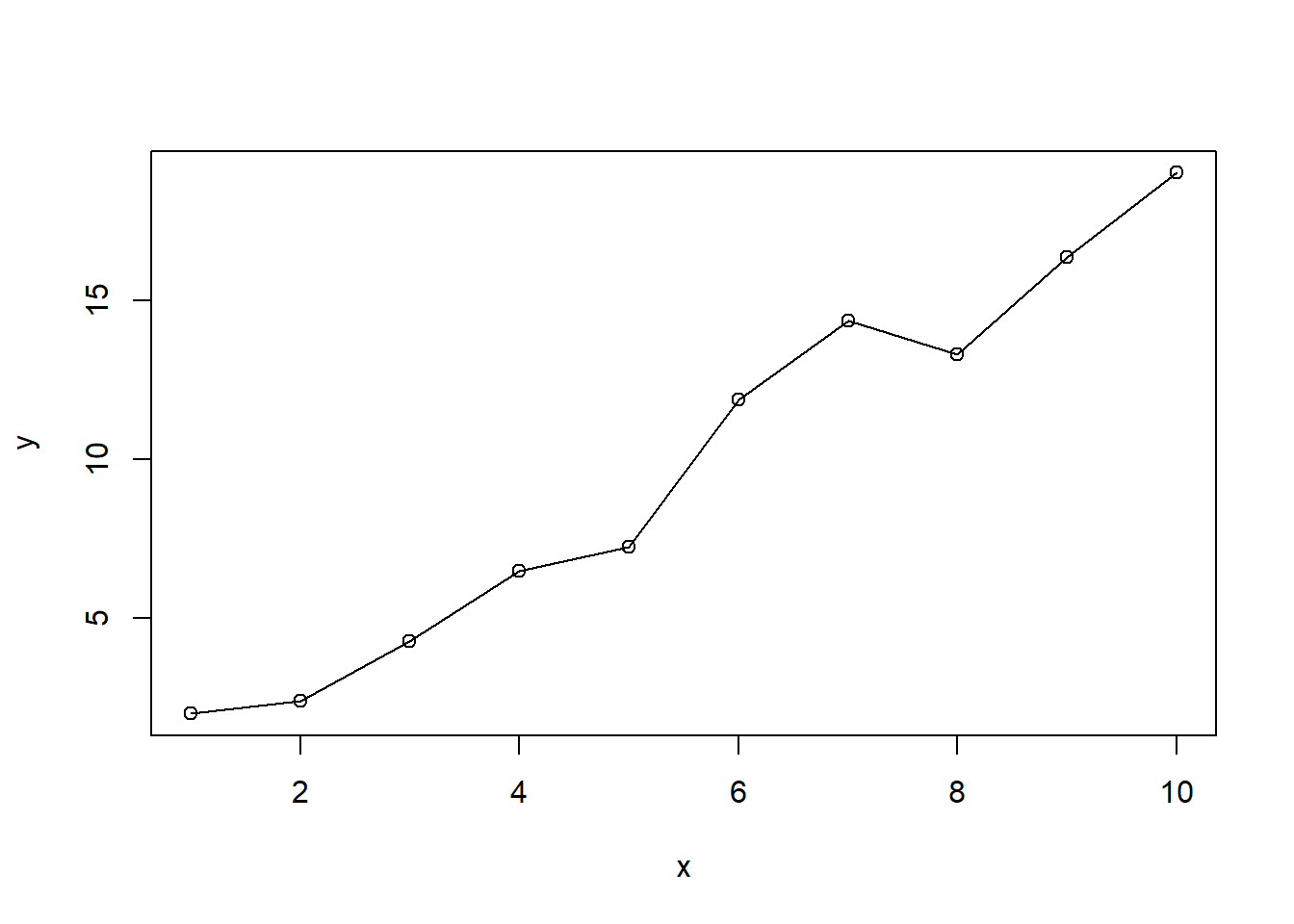# Under the hood: Maximum Likelihood Estimation (MLE)

Understand the working mechanism of one of the most used statistical methods.
Author

Murat Koptur

Published

October 1, 2022

# IntroductionSource: $$^1$$

Maximum likelihood is a method for constructing an estimator for an unknown parameter $$\theta$$, which was introduced by Fisher in 1912. Method can be applied in most problems and often gives a reasonable estimator for $$\theta$$, and if sample size is big, the method will give an excellent estimator of $$\theta$$. For these reasons, the method is most widely used estimation method in statistics $$^2$$.

Maximum likelihood estimation can be described as follow: suppose that we observed $$X_1,\cdots,X_n$$ from a distribution $$f(x|\theta)$$. Then we define the likelihood function (the joint probability of the observed data viewed as a function of parameters of the statistical model) as

$L(\theta)=f(x_1,\ldots,x_n|\theta)=f(x_1|\theta)\cdots f(x_n|\theta)$

Since $$\text{log}$$ is a monotonic increasing function, maximizing $$L(\theta)$$ is equivalent to maximizing $$\text{log}L(\theta)$$. Then we define the log-likelihood function as

$l(\theta)=\text{log}L(\theta)=\text{log}\prod_{i=1}^n f(X_i|\theta)=\sum_{i=1}^n\text{log}f(X_i|\theta)$

Maximizing $$l(\theta)$$ with respect to $$\theta$$ will give us the MLE $$^{2,3}$$:

$\hat{\theta}=\mathop{\mathrm{argmax}}_{\theta\in\Theta}l(\theta)$

# Examples

## Estimate the distribution parameters

Assume that we observed $$15$$ different $$X_i$$ values from Poisson distribution with unknown $$\lambda$$ (we simulate the data, so we know the true value of parameter $$\lambda = 3$$):

obs_pois <- rpois(15, 3)

plot(obs_pois)hist(obs_pois)Since we know that data is distributed as Poisson, we can write the likelihood and log-likelihood functions as:

$L(\lambda)=\prod_{i=1}^{n}\exp(-\lambda)\frac{1}{x_i!}\lambda^{x_i}$

$l(\lambda)=-n\lambda-\sum_{i=1}^n\ln(x_i!)+\ln(\lambda)\sum_{i=1}^nx_i$

Let’s estimate:

loglikMLEpois <- function(params, x_data) {
sum(dpois(x_data, lambda = params, log = TRUE))
}

estPois <- optim(c(lambda = 1), fn = loglikMLEpois, x_data = obs_pois, control = list(fnscale=-1), method="BFGS")

round(estPois$par, 4) lambda 2.9333  We estimated $$\hat{\lambda}=$$ 2.9333 which is a good estimation. Plot the log-likelihood function: logLikValuesPois <- expand.grid(lambda=seq(0, 10, 1)) logLikValuesPois$LL <- apply(logLikValuesPois, 1, loglikMLEpois, x_data=obs_pois)

plot(logLikValuesPois$lambda, logLikValuesPois$LL, type="o")## Estimate the linear regression model parameters

Suppose that we observed 10 different $$(x_i, y_i), \quad i=1,\ldots,10$$ samples. For this, we generate some random data (Our data generation process is $$y = 2x-1+\epsilon$$):

x <- seq(1, 10, 1)
y <- 2 * x - 1 + rnorm(10)

tibble(x, y)

Plot of our generated data:

plot(x, y, type="o")We simulated the data, so we know the true parameter values, $$\theta=(\theta_0, \theta_1)=(-1, 2)$$. Let’s try to estimate them with MLE.

If we assume that errors has the Normal distribution with mean zero and standard deviation one and error variance is constant, we get

$y~\sim N(\theta_0+\theta_1x,\sigma^2)$

So we can write the conditional distribution as:

$f(y|x,\theta_0,\theta_1,\sigma^2)=\frac{1}{\sqrt{2\pi\sigma^2}}e^{-\frac{1}{2}\frac{(y-(\theta_1x+\theta_0))^2}{\sigma^2}}$

Then we get following likelihood and log-likelihood functions:

$L(\theta_0,\theta_1,\sigma^2)=\frac{1}{(2\pi\sigma^2)^{n/2}}\prod_{(x,y)\in X}e^\frac{-(y-(\theta_1 x + \theta_0))^2}{2\sigma^2}$

$l(\theta_0,\theta_1,\sigma^2)=-\frac{n}{2}\log 2\pi-n\log \sigma-\frac{1}{\sigma^2}\sum_{i=1}^n(y_i-(\theta_1x_i+\theta_0))^2$

Let’s define log-likelihood function in R:

loglikMLEreg <- function(params, y_data) {
x_data = x
sum(dnorm(y_data, mean = params + params * x_data, sd = params, log = TRUE))
}

Maximize:

estReg <- optim(c(intercept = 0, slope = 1, sigma = 1), fn = loglikMLEreg,
y_data = y, control = list(fnscale=-1)
)

round(estReg$par, 4) intercept slope sigma -1.0947 1.9684 1.0153  Our estimation is $$\hat{\theta}=($$-1.0946921, 1.9683684$$)$$. We know that true values are $$\theta=(-1, 2)$$ so this estimation is a pretty good. Lastly, visualize our log-likelihood function with constant sigma: library(reshape2) library(plotly) logLikValues <- expand.grid(theta_0=seq(-5, 5, 1), theta_1=seq(-5, 5, 1)) logLikValues$sigma <- 1
logLikValues\$LL <- apply(logLikValues, 1, loglikMLEreg, y_data=y)

plot_matrix <- t(acast(logLikValues, theta_0~theta_1, value.var="LL"))

plot_ly(
x = as.numeric(colnames(plot_matrix)),
y = as.numeric(rownames(plot_matrix)),
z = plot_matrix
) %>%
layout(
scene = list(
xaxis = list(title = "theta_0"),
yaxis = list(title = "theta_1"),
zaxis = list(title = "LL")
))

Full source code: https://github.com/mrtkp9993/MyDsProjects/tree/main/MLE

## Citation

BibTeX citation:
@online{koptur2022,
author = {Murat Koptur},
title = {Under the Hood: {Maximum} {Likelihood} {Estimation} {(MLE)}},
date = {2022-10-01},
url = {https://www.muratkoptur.com/MyDsProjects/Analysis.html},
langid = {en}
}